Satterthwaite Degrees of Freedom Approximation

The DDFM=SATTERTHWAITE option in the MODEL statement requests denominator degrees of freedom intests andtests computed according to a general Satterthwaite approximation. The DDFM=KENWARDROGER option also entails the computation of Satterthwaite-type degrees of freedom.

The general Satterthwaite approximation computed in PROC GLIMMIX for the test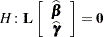is based on thestatistic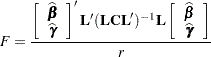where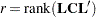, and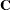is the approximate variance matrix of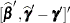; see the section Estimated Precision of Estimates and the section Aspects Common to Adaptive Quadrature and Laplace Approximation.

The approximation proceeds by first performing the spectral decomposition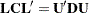, where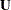is an orthogonal matrix of eigenvectors and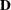is a diagonal matrix of eigenvalues, both of dimension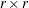. Define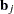to be theth row of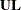, and let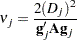where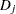is theth diagonal element ofand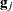is the gradient of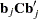with respect to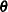, evaluated at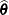. The matrix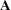is the asymptotic variance-covariance matrix of, obtained from the second derivative matrix of the likelihood equations. You can display this matrix with the ASYCOV option in the PROC GLIMMIX statement.

Finally, let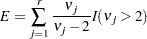where the indicator function eliminates terms for which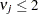. The degrees of freedom forare then computed as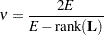provided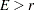; otherwise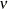is set to zero.

In the one-dimensional case, when PROC GLIMMIX computes atest, the Satterthwaite degrees of freedom for thestatistic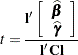are computed as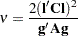where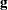is the gradient of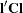with respect to, evaluated at.